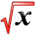# Related RatesThis article is part of the MathHelp Tutoring Wiki
• Q** Helium is pumped into a spherical balloon at a rate of 4 cubic feet per second. How fast is the radius increasing after 3 minutes? Note: The volume of a sphere is given by :$V=(4/3)\pi r^{3}$• A**: Derivative is ${\frac {dV}{dt}}=4\pi r^{2}{\frac {dr}{dt}}$. We are given $dV/dt=4m^{3}/s$. You can figure out the radius after 3 mins by volume which will be 3*4 or 12 cubic meters. Once we have the radius we can solve for dr/dt.